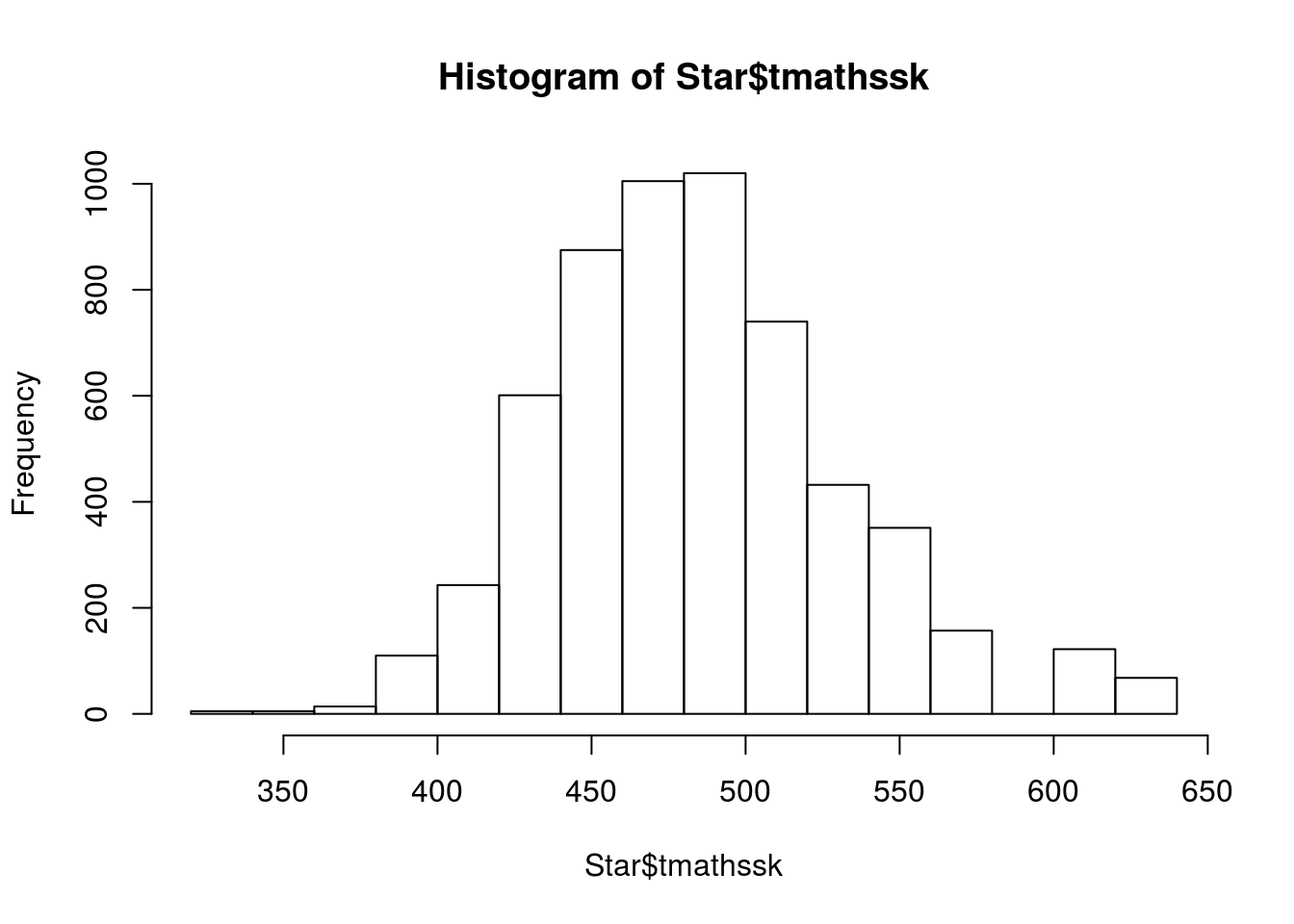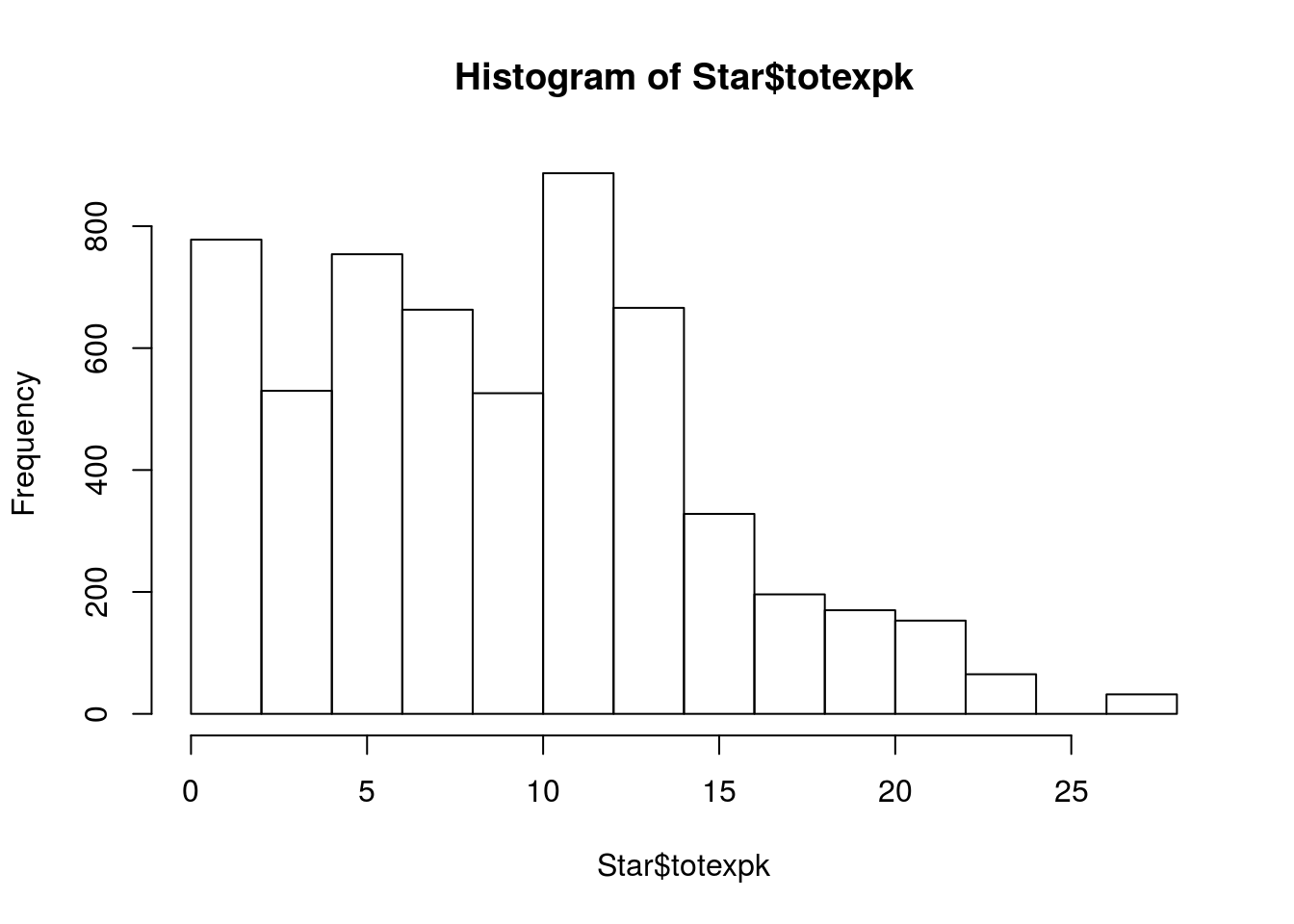# Linear Discriminant Analysis in R

In this post we will look at an example of linear discriminant analysis (LDA). LDA is used to develop a statistical model that classifies examples in a dataset. In the example in this post, we will use the “Star” dataset from the “Ecdat” package. What we will do is try to predict the type of class the students learned in (regular, small, regular with aide) using their math scores, reading scores, and the teaching experience of the teacher. Below is the initial code

``````library(Ecdat)
```library(MASS)
`data(Star)````

We first need to examine the data by using the “str” function

``str(Star)``
``````## 'data.frame':    5748 obs. of  8 variables:
##  \$ tmathssk: int  473 536 463 559 489 454 423 500 439 528 ...
##  \$ treadssk: int  447 450 439 448 447 431 395 451 478 455 ...
##  \$ classk  : Factor w/ 3 levels "regular","small.class",..: 2 2 3 1 2 1 3 1 2 2 ...
##  \$ totexpk : int  7 21 0 16 5 8 17 3 11 10 ...
##  \$ sex     : Factor w/ 2 levels "girl","boy": 1 1 2 2 2 2 1 1 1 1 ...
##  \$ freelunk: Factor w/ 2 levels "no","yes": 1 1 2 1 2 2 2 1 1 1 ...
##  \$ race    : Factor w/ 3 levels "white","black",..: 1 2 2 1 1 1 2 1 2 1 ...
##  \$ schidkn : int  63 20 19 69 79 5 16 56 11 66 ...
##  - attr(*, "na.action")=Class 'omit'  Named int [1:5850] 1 4 6 7 8 9 10 15 16 17 ...
##   .. ..- attr(*, "names")= chr [1:5850] "1" "4" "6" "7" ...``````

We will use the following variables

• dependent variable = classk (class type)
• independent variable = tmathssk (Math score)
• independent variable = totexpk (Teaching experience)

We now need to examine the data visually by looking at histograms for our independent variables and a table for our dependent variable

``hist(Star\$tmathssk)````hist(Star\$treadssk)````hist(Star\$totexpk)````prop.table(table(Star\$classk))``
``````##
##           regular       small.class regular.with.aide
##         0.3479471         0.3014962         0.3505567``````

The data mostly looks good. The results of the “prop.table” function will help us when we develop are training and testing datasets. The only problem is with the “totexpk” variable. IT is not anywhere near to be normally distributed. TO deal with this we will use the square root for teaching experience. Below is the code

``````star.sqrt<-Star
star.sqrt\$totexpk.sqrt<-sqrt(star.sqrt\$totexpk)
hist(sqrt(star.sqrt\$totexpk))``````Much better. We now need to check the correlation among the variables as well and we will use the code below.

``````cor.star<-data.frame(star.sqrt\$tmathssk,star.sqrt\$treadssk,star.sqrt\$totexpk.sqrt)
cor(cor.star)``````
``````##                        star.sqrt.tmathssk star.sqrt.treadssk
## star.sqrt.tmathssk             1.00000000          0.7135489
## star.sqrt.totexpk.sqrt         0.08647957          0.1045353
##                        star.sqrt.totexpk.sqrt
## star.sqrt.tmathssk                 0.08647957
## star.sqrt.totexpk.sqrt             1.00000000``````

None of the correlations are too bad. We can now develop our model using linear discriminant analysis. First, we need to scale are scores because the test scores and the teaching experience are measured differently. Then, we need to divide our data into a train and test set as this will allow us to determine the accuracy of the model. Below is the code.

``````star.sqrt\$tmathssk<-scale(star.sqrt\$tmathssk)
star.sqrt\$totexpk.sqrt<-scale(star.sqrt\$totexpk.sqrt)
train.star<-star.sqrt[1:4000,]
test.star<-star.sqrt[4001:5748,]``````

Now we develop our model. In the code before the “prior” argument indicates what we expect the probabilities to be. In our data the distribution of the the three class types is about the same which means that the apriori probability is 1/3 for each class type.

``````train.lda<-lda(classk~tmathssk+treadssk+totexpk.sqrt, data =
train.star,prior=c(1,1,1)/3)
train.lda``````
``````## Call:
## lda(classk ~ tmathssk + treadssk + totexpk.sqrt, data = train.star,
##     prior = c(1, 1, 1)/3)
##
## Prior probabilities of groups:
##           regular       small.class regular.with.aide
##         0.3333333         0.3333333         0.3333333
##
## Group means:
## regular           -0.04237438 -0.05258944  -0.05082862
## small.class        0.13465218  0.11021666  -0.02100859
## regular.with.aide -0.05129083 -0.01665593   0.09068835
##
## Coefficients of linear discriminants:
##                      LD1         LD2
## tmathssk      0.89656393 -0.04972956
## totexpk.sqrt -0.49061950  0.80051026
##
## Proportion of trace:
##    LD1    LD2
## 0.7261 0.2739``````

The printout is mostly readable. At the top is the actual code used to develop the model followed by the probabilities of each group. The next section shares the means of the groups. The coefficients of linear discriminants are the values used to classify each example. The coefficients are similar to regression coefficients. The computer places each example in both equations and probabilities are calculated. Whichever class has the highest probability is the winner. In addition, the higher the coefficient the more weight it has. For example, “tmathssk” is the most influential on LD1 with a coefficient of 0.89.

The proportion of trace is similar to principal component analysis

Now we will take the trained model and see how it does with the test set. We create a new model called “predict.lda” and use are “train.lda” model and the test data called “test.star”

``predict.lda<-predict(train.lda,newdata = test.star)``

We can use the “table” function to see how well are model has done. We can do this because we actually know what class our data is beforehand because we divided the dataset. What we need to do is compare this to what our model predicted. Therefore, we compare the “classk” variable of our “test.star” dataset with the “class” predicted by the “predict.lda” model.

``table(test.star\$classk,predict.lda\$class)``
``````##
##                     regular small.class regular.with.aide
##   regular               155         182               249
##   small.class           145         198               174
##   regular.with.aide     172         204               269``````

The results are pretty bad. For example, in the first row called “regular” we have 155 examples that were classified as “regular” and predicted as “regular” by the model. In rhe next column, 182 examples that were classified as “regular” but predicted as “small.class”, etc. To find out how well are model did you add together the examples across the diagonal from left to right and divide by the total number of examples. Below is the code

``(155+198+269)/1748``
``##  0.3558352``

Only 36% accurate, terrible but ok for a demonstration of linear discriminant analysis. Since we only have two-functions or two-dimensions we can plot our model.  Below I provide a visual of the first 50 examples classified by the predict.lda model.

``````plot(predict.lda\$x[1:50])
text(predict.lda\$x[1:50],as.character(predict.lda\$class[1:50]),col=as.numeric(predict.lda\$class[1:100]))
abline(h=0,col="blue")
abline(v=0,col="blue")``````The first function, which is the vertical line, doesn’t seem to discriminant anything as it off to the side and not separating any of the data. However, the second function, which is the horizontal one, does a good of dividing the “regular.with.aide” from the “small.class”. Yet, there are problems with distinguishing the class “regular” from either of the other two groups.  In order improve our model we need additional independent variables to help to distinguish the groups in the dependent variable.